## Wednesday, May 23, 2012

### Area of an Ellipse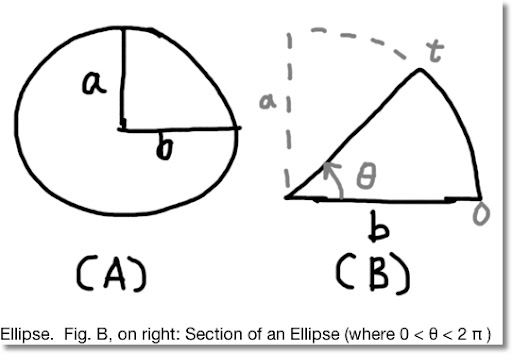Blog Entry #104. (I thought I use a different font this time. I like Arial, but change is good, at least every once in a while. )

Objective of this blog entry: calculate the area of an ellipse (Fig. A), and then a section of an ellipse (Fig. B).

Normally, the equation given for an ellipse is:

x^2/a^2 + y^2/b^2 = 1

I am going to use the parametric form

x = a cos t
y = b sin t
with 0 ≤ t ≤ 2 π

Let θ be the upper limit (angle) and the area is:

θ
∫ y dx =
0

θ
∫ y(t) x'(t) dt =
0

θ
∫ (b sin t) (a sin t) dt =
0

θ
∫ a b sin^2 t dt
0

Note: the antiderviatve of sin^2 t is 1/2 ( t - sin t cos t ).

Then:

θ
∫ a b sin^2 t dt =
0

a b [ 1/2 ( θ - sin θ cos θ ) - 1/2 ( 0 - sin 0 cos 0 ) ] =

1/2 a b ( θ - sin θ cos θ )

The area of an ellipse (up to angle θ , see Fig. B) is:
1/2 a b ( θ - sin θ cos θ )

To find the area of the entire ellipse, let θ = 2 π. Then:

A = 1/2 a b (2 π - sin (2 π) cos (2 π)) = π a b

That concludes is blog entry. Before I go, and before I forget: THANK YOU U.S. TROOPS FOR ALL YOU DO!!!!

Eddie

### Right Cylinders and Skewed Cylinders

Blog Entry # 103: One of my favorite things to do in mathematics is to find areas and volumes of unusual shapes.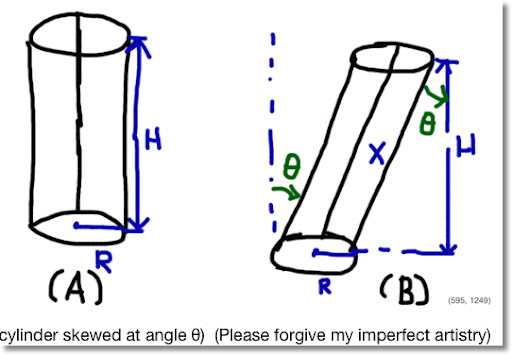Volume of a Right Cylinder (Figure A)

V = π R^2 H

H = height

Volume of a Skewed Cylinder at Angle θ (Figure B)

H resembles the measure from the base to the top of the skewed cylinder. However, to calculate the volume, we will need the length X, not H.

Using trigonometry:

cos θ = H / X

implies that

X = H / (cos θ)

The volume of the skewed cylinder is:

V = π R^2 H sec θ

Until next time, Eddie

## Monday, May 21, 2012

### Arc length of sin(x) - Curve Approximation

Blog Entry #102

The Arc Length of a Sine Curve

The sine curve is one the most interesting curves in mathematics.

Let

y = a * sin x ,

Where a is the amplitude of the sine curve.

We can find the arc length of a curve between limits x1 and x2 by the integral:

x2
∫ √ (1 + (dy/dx)^2) dx
x1

For the sine curve:

y = a sin x

dy/dx = a cos x

(dy/dx)^2 = a^2 cos^x

And the arc length is:

x2
∫ √(1 + a^2 cos^x) dx
x1

There is no anti-derivative for √(1 + a^2 cos^x). Therefore, numerical methods must be used.

Finding an Approximate Curve

Using a TI nSpire CX CAS, I used the Spreadsheet, curve fitting, and graphing features to determine an approximate polynomial. The arc length is from the origin (0,0) to (π, 0).

Note: x1 = 0, x2 = π

Here is a shot summary of what I did: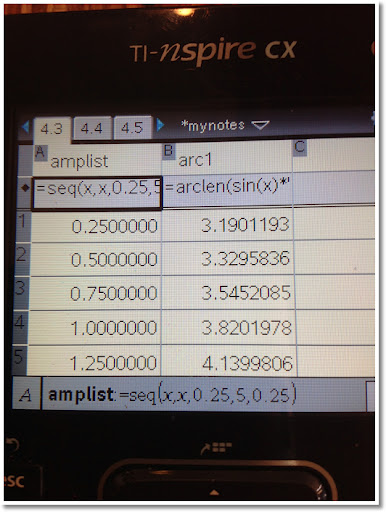1. Created a spreadsheet with the following columns:

Column A: A sequence of numbers from 0.25 to 5 in increments of 0.25. The resulting list is named amplist.

Column B: Use the nSpire's arcLen function to get the arc length of the sine curve using amplist as the values for a. This list is named arc1.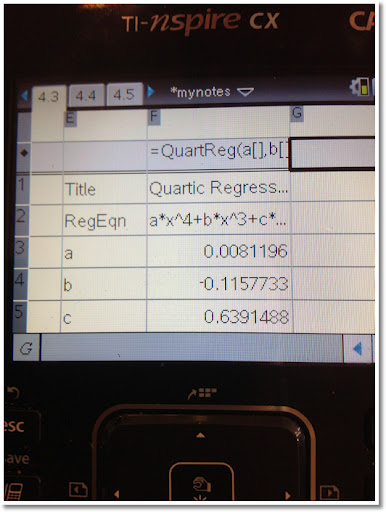2. Pressing the menu key allowed me to access the Statistics menu. Using the Stat Calculations option, I used different types of regression, including power and quartic regression. What I was looking for was which regression had the best coefficient of determination (R^2). In general, the closer R^2 is to 1, the better the fit.

I ended up choosing the quartic regression (4th degree polynomial) with R^2 ≈ 0.9999919.

The approximate polynomial is

y = .0081196317102889 x^4 - .11577326164517 x^3 + .63914882375794 x^2 + .2071162669684 x + 3.0881429428239

This polynomial was save to the function f1(x).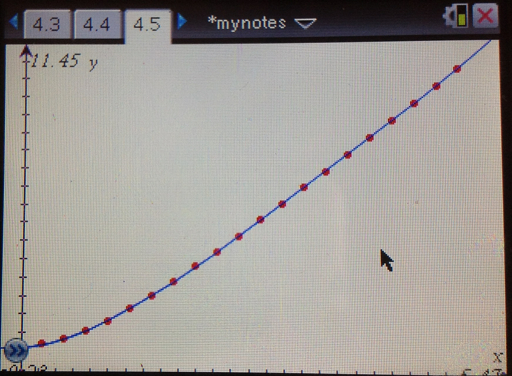3. I created a graphs page and made two plots:

* Scatter plot where x = amplist, y = arc1. (The dots in red)
* The function f1(x) (see step 2). (The curve in blue)

Setting zoom to fit the data, the curve looks like a good fit.

You can create a similar graph with the Data & Statstics module, but I thought I would be different this time.

How good of a fit is the polynomial?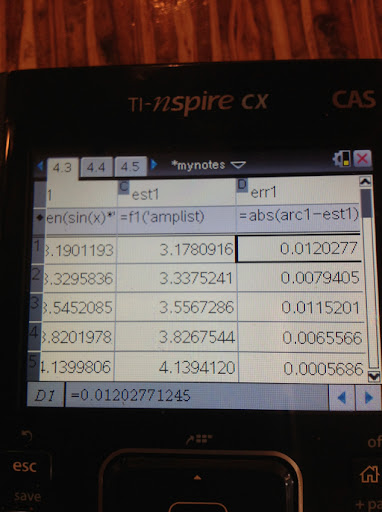4. I went back to the spreadsheet and added two more columns.

Column C: est1 = f1(amplist). (estimate arc lengths)
Column D: err1 = abs(arc1 - est1)

By scrolling down Column D, the quartic polynomial was accurate in estimating the arc length of the sine curve from 0 to π to at least two decimal places.

Conclusion

We have been looking to find the arc length of the curve y = a sin x from x = 0 to x = π.

The exact value is:

π
∫ √ (1 + a^2 cos^2 x ) dx
0

However, a good estimate can be found (to 2-3 decimal places) with the polynomial:

y = .0081196317102889 x^4 - .11577326164517 x^3 + .63914882375794 x^2 + .2071162669684 x + 3.0881429428239

Thanks as always and talk to you soon!

Eddie

This blog is property of Edward Shore. © 2012

## Thursday, May 17, 2012

### Financial Calculators: Finding a Balance

Blog Entry #101

The Balance of an Annuity

If you have a financial calculator, chances are that you have a Amortization function, which assists you in finding the interest and principal paid and the balance after a set of payments.

Another quick way way to find the balance is to calculate the future value (FV).

Example:

A 20-year loan for \$100,000 is issued at 5% annual interest. Find the monthly payment.

Known:
PV (Present Value) = 100,000
I/YR (Annual Interest) = 5%
Periodic Interest = 5/12 %
N (number of payments) = 20 * 12 = 240
FV (Future Value) = 0

Computed (HP 10bII+):
PMT (Payment) = -659.955739217 (-659.96 rounded to 2 places)

Two Ways to Find the Balance

Amortization Function

Enter the range of payments you want to amortize and execute the amortization function/spreadsheet.

For the HP 10bII+, to amortize the first k payments, press:

1 [INPUT] k [Down shift key] [FV] (AMORT)

The HP 10bII+ will display the range of payments to be amortized. Confirm this by pressing the equals key. [ = ].

The total principal paid is given. Press [ = ] to get the total interest paid. Press [ = ] one more time to get the balance.

In short, for the balance of the first n payments:
1 [INPUT] k [Down shift key] [FV] (AMORT) [ = ] [ = ] [ = ]

Future Value Method

To find the balance after the first k payments, enter k as N and compute future value (FV).

For example, if I want to find the balance of a loan after 12 payments, I enter 12 as N, and compute FV.

This is an alternate method, and a possible work-around for anyone having a finance calculator sans an amortization feature.

Remember: The TVM functions uses the cash-flow convention: positive numbers for receipts and negative numbers for payments.

A Comparison of Methods

A recap of the data in our example:

Known:
PV (Present Value) = 100,000
I/YR (Annual Interest) = 5%
Periodic Interest = 5/12 %
N (number of payments) = 20 * 12 = 240
FV (Future Value) = 0

Computed (HP 10bII+):
PMT (Payment) = -659.955739217 (-659.96 rounded to 2 places)

*Note: Signs will be ignored.

Balance after 60 payments:
Amortization Method: 83,454.57
FV Method: 83,454.86

Balance after 120 payments:
Amortization Method: 62,220.85
FV Method: 62,221.52

Balance after 180 payments:
Amortization Method: 34,970.35
FV Method: 34,971.35

I find similar results by using the Hewlett Packard HP 10bII+, Texas Instruments BA II Plus, and the Casio FC-200V.

Why is there a difference?

The TVM module uses a master equation involving N, I/YR, P/Y, PMT, PV, and FV and the financial calculator calls on this master equation to solve for the desired variable.

When using the amortization method, the financial calculator uses a different set of formulas. The formulas have this format:

Interest for the Period = Previous Balance * I/YR% * P/Y^-1

Principal for the Period = Payment - Interest for the Period

New Balance = Previous Balance - Principal for the Period

Prior to calculation, payment and interest are rounded.

For the exact formulas, consult the calculator financial manuals. Casio, Texas Instruments, and Hewlett Packard all provide formulas for TVM and amortization.

Link to the HP 10BII+ Manual: Check the Appendix

Thank you and take care!

Eddie

2012

## Thursday, May 10, 2012

Introduction

This blog entry will contain links to all 21 parts of the Calculus Revisited Series. Each link will open a new window.

**Some posts are out of order date wise - so please keep this page open if you want to go through the parts sequentially.

I hope this review is helpful to you, whenever you are studying for the final exam, want to review, or just want a refresher.

Thanks as always!

Eddie

Pages

#1: Functions
http://edspi31415.blogspot.com/2012/04/calculus-revisited-1-functions.html

#2: Trig Functions
http://edspi31415.blogspot.com/2012/04/calculus-revisited-2-working-with.html

#3: Logarithm and Exponential Functions
http://edspi31415.blogspot.com/2012/04/calculus-revisited-3-logarithmic-and.html

#4: Limits
http://edspi31415.blogspot.com/2012/04/calculus-revisited-4-limits.html

#5: Derivatives
http://edspi31415.blogspot.com/2012/04/calculus-revisited-5-derivatives.html

#6: Chain Rule
http://edspi31415.blogspot.com/2012/04/calculus-revisited-6-chain-rule.html

#7: Finding Extrema
http://edspi31415.blogspot.com/2012/04/calculus-revisited-7-finding-extrema.html

#8: Related Rates
http://edspi31415.blogspot.com/2012/04/calculus-revisited-8-related-rates.html

#9: Newton's Method
http://edspi31415.blogspot.com/2012/05/calculus-revisited-9-newton-method.html

#10: Integration Basics
http://edspi31415.blogspot.com/2012/04/calculus-revisited-10-integration.html

#11: Integration by Substitution
http://edspi31415.blogspot.com/2012/05/calculus-revisited-11-integration-by.html

#12: Integration by Parts
http://edspi31415.blogspot.com/2012/05/calculus-revisited-12-integration-by.html

#13: Integration Using Trig Identities
http://edspi31415.blogspot.com/2012/05/calculus-revisited-13-integrals.html

#14: Integration Using Partial Fractions
http://edspi31415.blogspot.com/2012/05/calculus-revisited-14-integration.html

#15: Infinite Limits
http://edspi31415.blogspot.com/2012/05/calculus-revisited-15-infinite-limits.html

#16: Improper Integrals
http://edspi31415.blogspot.com/2012/05/calculus-revisited-16-improper.html

#17: Sequences
http://edspi31415.blogspot.com/2012/05/calculus-revisited-17-sequences.html

#18: Series
http://edspi31415.blogspot.com/2012/05/calculus-revisited-18-series.html

#19: Tests of Convergence
http://edspi31415.blogspot.com/2012/05/calculus-revisited-19-tests-of.html

# 20: Taylor Series and Maclaurin Series
http://edspi31415.blogspot.com/2012/04/calculus-revisited-20-taylor-series-and.html

#21: The Catch All (Other Topics)
http://edspi31415.blogspot.com/2012/05/calculus-revisited-21-catch-all.html

### Calculus Revisited #21: The Catch All

Welcome to Part 21 of our 21 part series on Calculus Series. Here are some interesting facts about calculus that were not discussed in the first 20 parts, but are worth paying attention to:

Implicit Differentiation

The key here is that when you are differentiating, you differentiate with respect to the specific variable. All other variables are treated as constants.

So for the function f(x,y):

d/dx f(x,y) means differentiate with respect to x, y is held constant

d/dy f(x,y) means differentiate with respect to y, x is held constant

Example: Let f(x,y) = ln(x + 2y) + y^2

d/dx f(x,y)
= d/dx ln(x + 2y) + d/dx y^2
= 1/(x + 2y) + 0
= 1/(x + 2y)

d/dx f(x,y)
= d/dy ln(x + 2y) + d/dy y^2
= 2/(x + 2y) + 2y

Arc Length

The arc length of a function f(x) is given by the integral:

b
∫ √( 1 + (f'(x))^2 ) dx
a

Usually, you will be finding the arc length by numerical methods and/or the calculator.

A numerical example: The arc length of f(x) = sin x from x = 0 to x = π/2 (to seven decimal places)

f(x) = sin x
f'(x) = cos x
(f'(x))^2 = cos^2 x

Then:

π/2
∫ (1 + cos^2 x) dx ≈ 1.9100989 (by calcualtor)

A Numerical Method of Integration: Simpson's Rule

For n partitions (n is even):

b
∫ f(x) dx
a

≈ (b - a)/3n * (f(a) + 4*f(x1) + 2*f(x2) + 4*f(x3) + 2*f(x4) + ... + 4*f(x_2k+1) + 2*f(x_2k+2) + .... + 4*f(x_n-1) + f(b))

where x_k = a + (b - a)/n * k

Error:

At maximum (b - a)^5/(180 * (2n)^4) * max|f''''(x)|

In general, the more complex the integral, the more terms needed

Example:
Use the Simpsons rule to calculate

2
∫ e^x dx with n = 4 (7 decimal places)
1

(b - a)/n = (2 - 1)/4 = 1/4

The integral is approximately:

≈ (2 - 1)/(3 * 4) * (f(1) + 4 * f(1.25) + 2 * f(1.5) + 4 * (1.75) + f(2))
≈ 4.6708749

Area Between Curves

Let f(x) and g(x) be two functions where f(x) ≥ g(x). The area between curves f(x) and g(x) is:

b
∫ f(x) - g(x) dx
a

Often a and b will be intersection points of f(x) and/or g(x).

Example:

Let f(x) = x^2 and g(x) = x. Find the area between the curves from x = 0 to x = 5.

When 0 ≤ x ≤ 5, f(x) ≥ g(x). Then the area between the curves is:

5
∫ x^2 - x dx = 175/6 ≈ 29.1666667
0

Volume of a Solid - Disk Method

The volume of a solid of revolution - method of discs revolving around the x-axis is:

b
∫ π * (f(x))^2 dx
a

Like arc length, you may be finding the volumes numerically and/or by calculator (like I will do by this example).

Example: Let f(x) = sin x from x = 0 to x = π

Then the volume is:

π
∫ π * sin^2 x dx ≈ 4.9348022 = π^2/2
0

If you are finding a volume with discs revolving around the y-axis then the volume would be:

b
∫ π * (g(y))^2 dy
a

where a and b are y-values.

Polar Equations and Polar Integral

To convert functions to their polar form and back, use the following:

x = r cos θ
y = r sin θ

r^2 = x^2 + y^2
θ = atan (y/x)

The Polar Integral is:

θ2
∫ 1/2 * (r(θ))^2 dθ
θ1

Example: Let r(θ) = 2 θ . Find the polar integral from θ = 0 to θ = 2π

Area:

∫ 1/2 * (2 θ)^2 dθ = 16 π^3/ 3 ≈ 165.3668090
0

Parametric Equations

Parametric equations are in the form of ( x(t), y(t) ), where t is the independent variable and x and y are dependent variables. Each part can be separately differentiated and integrated.

Arc length of a parametric curve from t = a to t = b:

b
∫ √( (x'(t))^2 + (y'(t))^2 ) dt
a

Let:
x(t) = 2t
y(t) = t^3

Find (x, y) at t = 0 and t = 1. Find the arc length.

x(0) = 0, y(0) = 0
x(1) = 2, y(1) = 1

Arc length:

x'(t) = 2
y'(t) = 3t^2

(x'(t))^2 = 4
(y'(t))^2 = 9t^4

1
∫ √(4 + 9t^4) dt ≈ 2.3650656
0

Tips for Sketching a Curve y = f(x)

Find out where the extrema of f(x) are. Recall the critical points are found when:

f'(x) = 0

Let c be the critical points.

If f''(c) > 0, then x = c is strict minimum.

If f''(c) < 0, then x = c is a strict maximum.

If f'(c) > 0 when both f'(x) > 0 for x < c and x > c OR
f'(c) < 0 when both f'(x) < 0 for x < c and x > c,
then x = c is an inflection point.

When f'(x) > 0, f(x) is increasing.

When f'(x) < 0, f(x) is decreasing.

When f''(x) > 0, f(x) is concave up (holding water).

When f''(x) < 0, f(x) is concave down (spilling water).

If f(x) → +∞ or -∞ when x → a, then f(x) has a vertical asymptote at x = a.

If f(x) → b when x → +∞ or -∞, then f(x) has a horizontal asymptote at y = b.

Thank you as always. I hope you enjoyed the Calculus Revisited Series.

To all the students who are in calculus, good luck in finals!

Until next time,

Eddie

This blog is property of Edward Shore. © 2012

### Calculus Revisited #19: Tests of Convergence

Welcome to Part 19 of our 21 Part series: Calculus Revisited. We are in the middle of working with series. Today's session: Tests of Convergence.

In a calculus course, you are often asked if certain series converge - that is, does the series have a sum. You are not necessarily asked to find the sum itself, just to say if the series has one.

Hint: This is good to know, the p-series.

For a series

∑ 1/n^p
n = 1

this series converges when p > 1 and diverges when p ≤ 1.

Now for the tests:

Comparison Test:

Give the infinite series ∑ a(n), find another comparable infinite series ∑ b(n). The series used for comparison dominates the series in question. Or:

b_n ≥ a_n for each n an integer

In addition, all terms both series, ∑ a(n) and ∑ b(n), are positive.

If ∑ b(n) converges, so does ∑ a(n).

However, if ∑ a(n) diverges, so does ∑ b(n).

There is a variant of the comparison test call the limit test. That is if

lim ( a_n / b_n) = L as n → ∞,

If ∑ b(n) converges and L < ∞, ∑ a(n) converges.
If ∑ b(n) diverges and L > 0, ∑ a(n) diverges.

Ratio Test:

For a given infinite series ∑ a(n), if

lim | a_n+1 / a_n | = L as n → ∞, and

L < 1,

then ∑ a(n) is absolutely convergent. If L = 1, the test is inconclusive. If L > 1, the series diverges.

Note: A series is absolutely convergent if

∑ |a(n)| = | a(1) | + | a(2) | + | a(3) | + ....

is convergent.

If ∑ a(n) converges but ∑ | a(n) | does not, then the series is said to be conditionally convergent.

Root Test:

For a given infinite series ∑ a(n), if

lim | a_n |^(1/n) = L as n → ∞, and

L < 1,

then ∑ a(n) is absolutely convergent. If L = 1, the test is inconclusive. If L > 1, the series diverges.

Alternating Series Test:

For the existing series:

∑ (-1)^n * a(n),

if the sequence {a_n} (ignoring the (-1)^n) is a strictly decreasing sequence of positive numbers (that is a_n+1 < a_n for all n), and

lim a_n = 0 as n → ∞,

the the alternating series is (at least) conditionally convergent.

Problems

1. Does this series converge?

∑ n^2 / (2n - 1)!
n = 1

Use the ratio test. Then:

a_n = n^2 / (2n - 1)!

a_n+1 = (n + 1)^2 / (2(n + 1) - 1)! = (n + 1)^2 / (2n + 1)!

a_n+1 / a_n
= (n + 1)^2 / (2n + 1)! * (2n - 1)! / n^2
= (n + 1)^2 / (n^2 * (2n + 1)(2n))
= (n^2 + 2n + 1) / (4n^4 + 2n^3)

As n → ∞, a_n+1 / a_n → 0 < 1.

By the ratio test, this series converges.

Sometimes reasoning will be necessary.

2. Does this series converge?

∑ 1 / (n * n^(1/n))
n = 1

Note that:
1 / (n * n^(1/n))
= 1 / (n^1 * n^(1/n))
= 1 / (n^(1 + 1/n))

Compare the series to the harmonic series:

∑ 1/n
n = 1

where 1/n ≥ 1/(n^(1 + 1/n))

By Limit test:

lim ( 1/(n^(1 + 1/n)) / (1/n) ) as n → ∞
= lim ( n / n^(1+ 1/n) ) as n → ∞

As n grows large, 1 + 1/n → 1
Hence, n / n^(1 + 1/n) → n / n → 1

Since ∑ (1/n) diverges, so does ∑ 1/ (n * n^(1/n))

3. Does this series converge?

∑ 1/(4n - 3)
n = 1

Use the limit test. Compare it to the harmonic series, which is known to diverge, and:

1/n ≥ 1/(4n - 3)

lim ( (1/(4n - 3)) / (1/n) ) as → ∞
= lim ( n / (4n - 3) ) as → ∞
= 1/4 > 0

Hence, the series in question diverges.

4. Does this series converge?

1 - 1/√3 + 1/√5 - 1/√7 + .... + (-1)^n/√(2n+1) + ....

Use the Alternating Series test.

Let a_n = 1/√(2n+1)

First a_n strictly decreases. Second, a_n → 0 as n → ∞.

By alternating series, the series conditionally convergent.

5. Does this series converge?

1/(2 ln 2) - 1/(3 ln 3) + 1/(4 ln 4) - 1/(5 ln 5) + ... + (-1)^n/(n ln n) + ....

Use the Alternating Series Test

Let a_n = 1/(n ln n),

a_n strictly decreases and a_n → 0 as n → ∞.

By alternating series, the series is conditionally convergent.

Next time we work with Taylor Series.

See you soon!

Eddie

This blog is property of Edward Shore. © 2012

### Calculus Revisited #18: Series

Welcome to Part 18 of our wonderful 21 part of our Calculus Revisited Series. I hope you are enjoying this series. An announcement: Part 21 will be a "catch all" section - covering some of the topics we don't get to in detail in this series.

The next three blog entries will be about series. Today, the basics.

Series:

A series is a sum of terms in a sequence. The series can be finite or infinite. An infinite series is convergent (has a value) if the following is true:

∑ a_n = S
n=i

and S < ∞

Here are some famous series:

Arithmetic Series:

a + (a + d) + (a + 2d) + (a + 3d) + ...

Sum for a finite arithmetic series:

n-1
∑ a + k * d
k=0

= a * n + (d * n * (n -1))/2

Geometric Series:

a + a * r + a * r^2 + a * r^3 + ...

Sum for an infinite geometric series, provided that |r| < 1:

∑ a * r^k = a / (1 - r)
k = 0

Harmonic Series:

∑ 1/n
n=1

= 1 + 1/2 + 1/3 + 1/4 + 1/5 + ....

There is no closed formula for a finite harmonic series. In addition the infinite harmonic series diverges (has no sum).

Series Properties:

∑ c * a(n) = c * ∑ a(n) (c is a constant)

∑ a(n) + b(n) = ∑ a(n) + ∑ b(n)

n
∑ k = n * (n + 1)/2
k=1

n
∑ k^2 = n * (n + 1) * (2n + 1)/6
k = 1

n
∑ k^3 = n^2 *(n + 1)^2 / 4
k = 1

Subtracting from a whole:
If t > 1:

k
∑ a(n) =
n = t

∑( a(n) for n = 1 to k) - ∑( a(n) for n = 1 to t - 1)

Problems

1. Calculate

6
∑ 50 - 2k
k = 0

This is an arithmetic series with n = 6 + 1 = 7, a = 50, and d = -2.

Then the sum is:
(50)(7) + (-2 * 7 * 6)/2 = 308

2. Show why the (infinite) harmonic series is divergent.

Here is one way:

Let S be the sum of the harmonic series, that is:

S = 1 + 1/2 + 1/3 + 1/4 + 1/5 + 1/6 + 1/7 + 1/8 + ...

Note that:
1 + 1/2 > 2/2
1/3 + 1/4 > 2/4
1/5 + 1/6 > 2/6
1/7 + 1/8 > 2/8
and so on...

Then we have 2/2 + 2/4 + 2/6 + 2/8 + ....

Which simplifies to 1 + 1/2 + 1/3 + 1/4 + .... = S

Which implies S > S, which is impossible.

The harmonic series is divergent.

3. Find the sum:

∑ .5^n + .3^n
n=0

We can first break the sum up:

∑(.5^n for n=0 to ∞) + ∑(.3^n for n=0 to ∞)

Both terms are geometric series. r = .5 for the first term and r = .3 for the second term.

Then:

∑(.5^n for n=0 to ∞) + ∑(.3^n for n=0 to ∞)
= 1 / (1 - .5) + 1 / (1 - .3)
= 1 / .5 - 1 / .7

4. Find the sum:

∑ 2 / (5^n)
n = 1

This looks like a geometric series with r = 1/5. If we can put the sum into it's proper form, perhaps by adding and subtracting 2 / 5^0:

∑( 2 / (5^n) for n = 1 to ∞)
= ∑ ( 2 / (5^n) for n = 0 to ∞) - 2 / 5^0
= ∑ ( 2 / (5^n) for n = 0 to ∞) - 2
= 2 / ( 1 - 1/5) - 2
= 2 / (4/5) - 2
= 5/2 - 2 = 1/2

5. Find the sum:

20
∑ k^2
k=10

Again, looks like a k^2 series, but use the subtraction from the whole technique and:

∑( k^2 for k = 10 to 20)
= ∑(k^2 for k = 1 to 20) - ∑(k^2 for k = 1 to 10 - 1)
= (20)(20 + 1)(2 * 20 + 1)/6 - (9)(9 + 1)(2 * 9 + 1)/6
= 17220 / 6 + 1710 / 6
= 15510 / 6 = 2585

Thank you once again for joining us. Next time we will work some series convergence tests.

Eddie

This blog is property of Edward Shore. © 2012

## Wednesday, May 9, 2012

### Calculus Revisited #17: Sequences

This Part 17 of the Calculus Revisited series. Today we are dealing with sequences.

Sequence:

A sequence of numbers is exactly what you think of, a list of numbers. An infinite sequence is a list of numbers that goes on forever. In calculus, it is understood that unless specified, a sequence is an infinite one.

{a_n} = a_1, a_2, a_3, .... , a_n; n is an integer

Convergent Sequence:

A sequence {a_n} converges when

lim a_n = L
n → ∞

when L < ∞.

If the above condition is not met, then the sequence is said to diverge.

Bounded Sequence:

A sequence {a_n} is bounded if there exists a C such that

-C ≤ a_N ≤ C (alternatively |a_n| ≤ C)

If the above condition is not true, then the sequence is said to be unbounded.

Problems

1. Write the first five terms of the sequence {a_n} = 1 / n^3. Does this sequence converge?

a_1 = 1 / 1^3 = 1
a_2 = 1 / 2^3 = 1/8
a_3 = 1 / 3^3 = 1/27
a_4 = 1 / 4^3 = 1/64
a_5 = 1 / 5^3 = 1/125

lim 1 / n^3 = 0 as n → ∞. Conclude that the sequence converges.

Note that the sequence is also bounded. | 1 / n^3 | ≤ 1

2. Write the first five terms of {a_n} = (-1)^(n-1) * n^2. Does this sequence converge.

a_1 = (-1)^(1-1) * 1^2 = 1
a_2 = (-1)^(2-1) * 2^2 = -4
a_3 = (-1)^(3-1) * 3^2 = 9
a_4 = (-1)^(4-1) * 4^2 = -16
a_5 = (-1)^(5-1) * 5^2 = 25

lim (-1)^(n-2) * n^2 as n → ∞:

(-1)^(n-2) is bounded between -1 and 1.
However, n^2 grows to infinity.

Hence (-1)^(n-2) * n^2 → ∞ as n → ∞, the sequence diverges.

Also the sequence is unbounded.

3. Find the first six terms of the sequence {a_n} defined by the recursion formula:

a_0 = 1; a_n = 3 * a_n-1 + 2

a_0 = 1
a_1 = 3 (0) + 2 = 2
a_2 = 3 (2) + 2 = 8
a_3 = 3 (8) + 2 = 26
a_4 = 3 (26) + 2 = 80
a_5 = 3 (80) + 2 = 242
a_6 = 3 (242) + 2 = 728

Coming up: Series!

Eddie

This blog is property of Edward Shore. © 2012

## Tuesday, May 8, 2012

### Calculus Revisited #16: Improper Integrals

Welcome to Part 16 of our 21 part Calculus Revisited series. Today we got down, dirty, and improper.

Improper Integrals

(I)

∫ f(x) dx =
a

lim ( ∫(f(x) dx, a, t) as t → ∞

If f(r) is undefined:

(II)

a
∫ f(x) dx =
r

lim ( ∫f(x) dx, t, a) as t → r+ (t approaches r from the right side)

(III)

r
∫ f(x) dx =
a

lim ( ∫(f(x) dx, a, t) as t → r- (t approaches r from the left side)

Problems

1. Calculate:

4
∫ 2/(x - 2) dx
2

Note 2/(x - 2) is defined at x = 2. This is an improper integral.

∫( 2/(x - 2) dx, 2, 4)
= lim ∫( 2/(x - 2) dx, t, 4) as t → 2+
= lim (2 ln(4 - 2) - 2 ln(t - 2)) as t → 2+

Note: ln t has no limit as t → 0

Therefore the integral has no finite answer. Yes, that can happen.

2. Calculate:

3
∫ dx / √(3 -x)
0

Note that 1/√(3 - x) is undefined at x = 3. Another improper integral.

∫( 1 / √(3 - x) dx, 0, 3)
= lim ∫( 1 / √(3 - x) dx, 0, t) as t → 3-
= lim (- 2 * √(3 - t) + 2 * √(3 - 0) ) as t → 3-
= lim ( -2 * √(3 - t) + 2√3) as t → 3-
= 2√3 ≈ 3.46410

3. Calculate:

∫ e^-x/2 dx
0

So:
∫ (e^(-x/2) dx, 0, ∞)
= lim (e^(-x/2) dx, 0, t) as t → ∞
= lim (-1/2* e^(-t/2) + 1/2 * e^0 ) as t → ∞
= 0 + 1/2
= 1/2

Next time we are going to work with sequences.

Until then, have a great day!

Eddie

This blog is property of Edward Shore. © 2012

## Monday, May 7, 2012

### Calculus Revisited #15: Infinite Limits

We have arrived at the 15th blog entry of the 21 entry series of Calculus Revisited. It is all about infinity today, and some quick tips with deal with infinity and limits.

Infinite Limits

General Rules for Polynomials

Let p(x) be a polynomial with degree n. The lead term of p(x) is a_n*x^n.

If n is even and a_n is positive:
* p(x) →+∞ as x → +∞
* p(x) → +∞ as x → -∞

If n is even and a_n is negative:
* p(x) → -∞ as x → +∞
* p(x) → -∞ as x → -∞

If n is odd and a_n is positive:
* p(x) → +∞ as x → +∞
* p(x) → -∞ as x → -∞

If n is odd and a_n is negative:
* p(x) → -∞ as x → +∞
* p(x) → +∞ as x → -∞

Generally: 1 / p(x) → 0 as x → +∞ or x → -∞

If p(x)/q(x) have the same degree, then

as x → +∞ or x → -∞

General Rules for Other Functions

ln x → -∞ as x → -∞
ln x → +∞ as x → +∞

e^x → 0 as x → -∞
e^x → +∞ as x → +∞

e^-x → +∞ as x → -∞
e^-x → 0 as x → +∞

sin x has no limit as x → +∞ or x → -∞

cos x has no limit as x → +∞ or x → -∞

tan x has no limit as x → +∞ or x → -∞

Note that sin x and cos x are both bounded between -1 and 1.

L'Hospital's Rule

For indeterminate forms 0/0, +∞/+∞, -∞/-∞ you can use the rule:

lim f(x)/g(x) =
x → a

lim f'(x)/g'(x)
x → a

Let's go over some problems.

Problems

1. Find: lim (1/x * sin) as x → ∞

We know that:

lim 1/x = 0 as x → ∞ and sin x is bounded.

Then the overall limit is 0 * (a number between -1 and 1) = 0

2. Find lim (e^(-x^2)) as x → ∞

In general, e^-x → 0 as x → ∞

Even though x^2 grows, e^-x shrinks faster and is dominant.

Hence lim (e^(-x^2)) = 0 as x → ∞.

The next three problems uses the p(x)/q(x) rules.

3. lim (x^2 + 1)/(x - 2) as x → ∞

Degree of numerator = 2
Degree of denominator = 1

Numerator will grow faster than the denominator.

Then lim (x^2 + 1)/(x - 2) → ∞ as x → ∞

4. lim (x^2 + 1)/(x^2 - 1) as x → ∞

Degree of numerator = 2
Degree of denominator = 2

Then lim (x^2 + 1)/(x^2 - 1) = 1/1 = 1 as x → ∞

5. lim (x^2 + 1)/(x^3 - 1) as x → ∞

Degree of numerator = 2
Degree of denominator = 3

Denominator will faster than numerator.

Then lim (x^2 + 1)/(x^3 - 1) = 0 as x → ∞

The next two problems uses L'Hospital's Rule.

6. lim (x^2 / e^x) as x → ∞

lim (x^2 / e^x) as → ∞ = ∞/∞ (Indeterminate form)

Applying the rule:

lim (2x / e^x ) as x → ∞ = ∞/∞ (Indeterminate form)

One more time:

lim (2 / e^x) as x → ∞ = 0

Therefore, lim (x^2 / e^x) = 0 as x → ∞

7. lim (sin x / x) as x → 0

lim (sin x / x) as x → 0 = 0/0 (Indeterminate form)

Applying the rule:

lim (cos x/1) as x→ 0 = 1/1 = 1

Therefore, lim (sin x / x) = 1 as x → 0

This concludes today's session. Next time we work with improper integrals (yes, there are such things!).

Have a great day,

Eddie

This blog is property of Edward Shore. © 2012

## Sunday, May 6, 2012

### The Integral of ∫ √x * √(1-x) dx

I was recently asked by Mike Grigsby to integrate:

∫ √x √(1-x) dx

-----
Make the substitution:

x = sin^2 θ

Note:

√x = sin θ ,
θ = asin x ,
dx = 2 sin θ cos θ dθ ,
and
cos θ = √(1 - sin^2 θ )
-----

Hence:

∫ √x √(1-x) dx (x to θ)
= ∫ sin θ * √(1 - sin^2 θ )* 2 *sin θ * cos θ dθ
= 2 ∫ sin^2 θ cos^2 θ dθ
= 2 ∫ (1 - cos^2 θ ) * cos^2 θ dθ
= 2 ∫ cos^2 θ - cos^4 θ dθ
= 2 ∫ 1/8 - 1/8 * cos (4θ) dθ (See Note A)
= 2 ( θ/8 - 1/8 * 1/4* sin (4θ))
= 2 ( θ/8 - 1/32 * sin (4θ))
= 2 ( θ / 8 - 1/32 * [8 sin θ cos^3 θ - 4 sin θ cos θ]) (See Note B)
(θ to x)
= 2 ( asin √x / 8 - 1/32 * [8 √x (1 - x)^3/2 - 4 √x √(1-x)] )
= 2 ( asin √x / 8 - (√x √(1-x) (2 - 2x - 1))/8 )
= asin √x / 4 - (√x √(1-x) (1 - 2x)) / 4

Final:

∫ √x √(1-x) dx = 1/4 * ( asin √x - (√x √(1-x) (1 - 2x)) + C
for some constant C

-----
Note A

cos 2a = 2 cos^2 a - 1
cos^2 a = 1/2 * (cos 2a + 1)

cos^4 a
= [1/2 * (cos 2a + 1)]^2
= 1/4 * (cos^2 2a + 2 cos 2a + 1)
= 1/4 * ( (cos 4a + 1)/2 + 2 cos 2a + 1)
= 1/8 * (cos 4a + 4 cos 2a + 3)
= 1/8 * cos 4a + 1/2 * cos 2a + 3/8

cos^2 a - cos^4 a
= (1/2 * cos 2a + 1/2) - (1/8 * cos 4a + 1/2 * cos 2a + 3/8)
= 1/8 - 1/8 * cos 4a
-----

-----
Note B

sin 2a = 2 sin a cos a

sin 4a
= 2 sin 2a cos 2a
= 8 sin a cos^3 a - 4 sin a cos a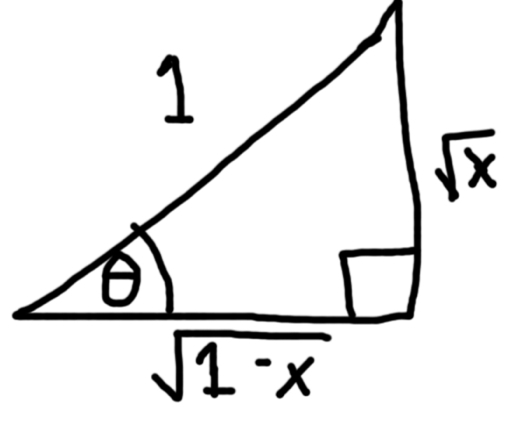Using the triangle from above:

sin θ = √x
cos θ = √(1-x)
-----

This blog is property of Edward Shore. © 2012

## Thursday, May 3, 2012

### Calculus Revisited #14: Integration - Decomposition of Fractions

Welcome to Part 14 of our 21 part of our Calculus Revisited. Today's blog entry involves integration using decomposition of fractions.

Decomposition of Fractions

Here is the general strategy.

If the denominator has (x - c)^r then:

α / (x - c)^r = A1 / (x - c) + A2 / (x - c)^2 + ... + Ar / (x - c)^r

If the denominator has (x^2 + cx + d)^r then:

β / (x^2 + cx + d) = (A1x + B1) / (x^2 + cx + d) + (A2 x + B2) / (x^2 + cx + d)^2 + ... + (Ar x + Br) / (x^2 + cx + d)^r

Here are some examples.

Problems

Sometimes polynomial division is all you need.

1. ∫ (8x - 3) / (4x + 1) dx

First execute the division (8x - 3) / (4x + 1).

(8x - 3) / (4x + 1) = 2 - 5 / (4x + 1)

So

∫ (8x - 3) / (4x + 1) dx
= ∫ 2 - 5 / (4x + 1) dx
= 2x - 5/4 * ln(4x + 1)

Final: ∫ (8x - 3) / (4x + 1) dx = 2x - 5/4 * ln(4x+ 1) + C

Now here are two basic examples of decomposition of fractions.

2. ∫ dx / ((x + 1)(2x + 3))

Then:

A / (x + 1) + B / (2x + 3 ) = 1 / ((x + 1)(2x + 3))

A (2x + 3) + B (x + 1) = 1

2A(x) + 3A + B(x) + B = 1 + 0(x)

Look at the coefficients and match them.

Constant: 3A + B = 1
Coef. of x: 2A + B = 0

The next task is to solve for A and B.

3A + B = 1
2A + B = 0

Subtract the second equation from the first equation

A = 1

Find B

Using the first equation:
3(1) + B = 1
B = 1 -3
B = -2

Using the second equation:
2(1) + B = 0
2 + B = 0
B = -2

Conclude A = 1, B = -2

Back to our integral:

∫ dx / ((x + 1)(2x + 3))
= ∫ A / (x + 1) + B / (2x + 3) dx
= ∫ 1 / (x + 1) - 2 / (2x + 3) dx
= ln(x + 1) - ln(2x + 3)
= ln( (x + 1) / (2x + 3) )

Final: ∫ dx / ((x + 1)(2x + 3)) = = ln( (x + 1) / (2x + 3) ) + C

3. ∫ (2x + 1) / ( (x^2 + 1) (x - 1) ) dx

Decomposing:

(2x + 1) / ( (x^2 + 1) (x - 1) ) = (Ax + B) / (x^2 + 1) + C / (x - 1)

Then:

(Ax + B) (x - 1) + C (x^2 + 1) = 2x + 1
A(x^2) - A(x) + B(x) - B + C(x^2) + C = 2x + 1 + 0(x^2)
(A + C)x^2 + (-A + B)x + (-B + C) = (0)x^2 + (2)x + 1

Matching up terms of x^2, x, and the constant:
A + C = 0
-A + B = 2
-B + C = 1

From the first equation, C = -A (alternatively A = -C)

Using the second and third equations:
-A + B = 2
-B + C = 1

-A + B = 2
-B + (-A) = 1

-A + B = 2
-A - B = 1

Add the two equations together to get

-2A = 3
A = -3/2

Then: -A - B = 1
-(-3/2) - B = 1
- B = 1 - 3/2
B = 3/2 - 1
B = 1/2

So A = -3/2, B = 1/2, and C = 3/2

And:
∫ (2x + 1) / ( (x^2 + 1) (x - 1) ) dx
= ∫ (Ax + B) / (x^2 + 1) + C / (x - 1) dx
= ∫ ((-3/2)x + 1/2) / (x^2 + 1) + (3/2) / (x - 1) dx
= ∫ (-3/2)x / (x^2 + 1) + (1/2) / (x^2 + 1) + (3/2) / (x - 1) dx
= (-3/4) ln(x^2 + 1) + (1/2) atan x + (3/2) ln (x - 1)

Final:
∫ (2x + 1) / ( (x^2 + 1) (x - 1) ) dx = (-3/4) ln(x^2 + 1) + (1/2) atan x + (3/2) ln (x - 1) + C

That concludes our integration techniques section. Next time we move to infinite limits.

Until next time, take care,

Eddie

This blog is property of Edward Shore. © 2012

### Calculus Revisited #13: Integrals Involving Trigonometric Identities

We are at Part 13 of a 21 part series. This time we will work with integrals involving trigonometric identities.

Problems
All problems today will be indefinite integrals. Problems with definite integrals will be handled similarly.

Identities Involving
sin^2 x + cos^2 x = 1
cos (2x) = 1 - 2 sin^2 x
sin (2x) = 2 sin x cos x
sin^2 x = (1 - cos 2x)/2
cos^2 x = (1 + cos 2x)/2

1. ∫ cos^2 x dx

∫ cos^2 x dx
= ∫ 1 - sin^2 x dx
= ∫ 1 dx - ∫ sin^2 x dx
= x - ∫ 1/2 - 1/2 * cos 2x dx
= x - ( ∫ 1/2 dx - ∫ 1/2 * cos 2x dx )
= x - 1/2* x + 1/2 * ∫ cos 2x dx
= 1/2 * x + 1/2 * sin 2x * 1/2
= 1/2 * x + 1/4 * sin 2x

Final: ∫ cos^2 x dx = 1/2 * x + 1/4 * sin 2x + C

Note:
∫ cos (ax) dx = sin (ax)/a
∫ sin (ax) dx = -cos (ax)/a

2. ∫ sin^2 x cos^2 x dx

∫ sin^2 x cos^2 x dx
= ∫ (1 - cos 2x)/2 * (1 + cos 2x)/2 dx
= ∫ 1/4 * (1^2 - cos^2 (2x)) dx
= 1/4 * ( ∫ 1 - cos^2 (2x) dx )
= 1/4 * ( ∫ 1 - (1 + cos 4x)/2) dx )
= 1/4 * ( ∫ 1/2 - 1/2 * cos 4x dx)
= 1/4 * (1/2 * x - 1/8 * sin 4x)

Final: ∫ sin^2 x cos^2 x dx = 1/4 * (1/2 *x - 1/8 * sin 4x) + C

Trigonometric Substitutions
If the integrand has...

√(a^2 - x^2): use the substitution x = a sin u, dx = a cos u du

√(a^2 + x^2): use the substitution x = a tan u, dx = a sec^2 u du

√(x^2 - a^2): use the substitution x = a sec u, dx = a tan u sec u du

These can get messy!

Identity: 1 + tan^2 x = sec^2 x

3. ∫ 1 / √(4 - x^2) dx

a^2 = 4
a = 2
x = 2 sin u
dx = 2 cos u du

∫ 1/√(4 - x^2) dx
= ∫ (2 cos u) / √(4 - 4 sin^2 u) du
= ∫ (2 cos u) / (√4 * √(1 - sin^2 u) ) du
= ∫ (2 cos u) / (2 * √( cos^2 u ) ) du
= ∫ (2 cos u) / (2 * cos u) du
= ∫ 1 du
= u

x = 2 sin u implies that u = asin(x/2)

= asin(x/2)

Final: ∫ 1 / √(4 - x^2) dx = asin(x/2) + C

4. ∫ 1/ √(x^2 + 64) dx

a^2 = 64
a = 8
x = 8 tan u
dx = 8 sec^2 u du
u = atan(x/8)

∫ 1/ √(x^2 + 64) dx
= ∫ (8 sec^2 u)/(√(64 * tan^2 u + 64) du
= ∫ (8 sec^2 u)/(8 * √(sec^2 u)) du
= ∫ (8 sec^2 u)/(8 * sec u) du
= ∫ sec u du

∫ sec x dx = ln |sec x + tan x|

= ln |sec u + tan u|
= ln | sec(atan(x/8)) + tan(atan(x/8)) |

Refer to the right triangle below: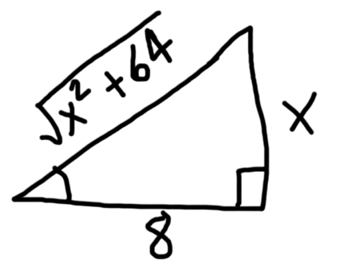u = atan (x/8)

tan u = x/8

cos u = 8/√(x^2 + 64)

sec u = √(x^2 + 64)/8

Then:

ln | sec(atan(x/8)) + tan(atan(x/8)) |
= ln | √(x^2 + 64)/8 + x/8 |
= ln | √(x^2 + 64) + x | - ln 8

Final:
∫ 1 / √(x^2 + 64) dx
= ln | √(x^2 + 64) + x | - ln 8 + C

Note that ln 8 + C is a constant. Then.

∫ 1 / √(x^2 + 64) dx
= ln | √(x^2 + 64) + x | + C

5. ∫ 1 / (x^2 - 25)^(3/2) dx

a^2 = 25
a = 5
x = 5 sec u
u = asec(x/5) = acos(5/x)
dx = 5 sec u tan u du

1 + tan^2 x = sec^ x

Then:
∫ 1 / (x^2 - 25)^(3/2) dx
= ∫ (5 sec u tan u) / ( 25 sec^2 u - 25 )^(3/2) du
= ∫ (5 sec u tan u) / ( 25 tan^2 u )^(3/2) du
= ∫ (5 sec u tan u) / ( 25^(3/2) * tan^3 u ) du
= 5 / 25^(3/2) * ∫ (sec u tan u) / tan^3 u du
= 1/25 * ∫ cos u/sin^2 u du
= 1/25 * ∫ cot u csc u du

∫ cot x csc x dx = -csc x

5 / 25^(3/2) = 5 / ((5^2)^(3/2)) = 5 / (5^3) = 1 / 5^2 = 1/25

= 1/25 * -csc u
= -1/25 * csc(acos(5/x))

Refer to the triangle below: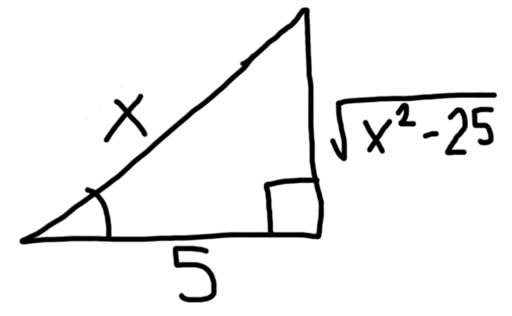x = 5 sec u

x/5 = sec u

5/x = cos u

√(x^2 - 25)/x = sin u

x/√(x^2 - 25) = csc u

Then:

-1/25 * csc(acos(5/x))
= -1/25 * x/√(x^2 - 25)

Final:
∫ 1 / (x^2 - 25)^(3/2) dx = -1/25 * x / √(x^2 - 25) + C

That concludes Part 13 of our series. Next time we will work with decomposition of fractions.

Have a good day,

Eddie

This blog is property of Edward Shore. © 2012

### Calculus Revisited #12: Integration by Parts

Welcome to Part 12 of our 21-part Calculus Revisited series. Today we will cover Integration by Parts in our second of four integration techniques.

Integration by Parts

∫ u(x) * v'(x) dx = u(x) * v(x) - ∫ v(x) * u'(x) dx

Pick the parts u(x) and v'(x) dx. Find the derivative of u(x) and the integral of v'(x).

The trick is to pick the parts u(x) and v'(x) so that v(x) * u'(x) is easier to integrate than u(x) * v'(x).

In practice, you may find that somewhere down the line, u(x) * v'(x) = v(x) * u'(x). This comes up especially where you have sines and cosines.

You may have to repeat this procedure more than once. Let's get to some examples.

Problems

The key to this technique is to pick the correct parts. Again, practice, practice, practice.

If you can pick u(x) such that du/dx is a constant, or goes towards a constant, it will most likely help.

Indefinite Integrals

1. ∫ x sin x dx

u = x
dv = sin x dx

du = 1 dx (take the derivative of u(x))
v = - cos x (take the integral of v(x))

Putting it together:

∫ x sin x dx
= (x) * ( - cos x ) - ∫ (- cos x) (1) dx
= - x * cos x + ∫ cos x dx

The second term is easy to integrate.

= -x * cos x + sin x

For indefinite integrals, you can tack on the "C" at the end

Final Answer: ∫ x sin x dx = -x* cos x + sin x + C

2. ∫ x * 3^x dx

u = x
dv = 3^x dx

du = 1 dx
v = 3^x / ln 3

Then:

∫ x * 3^x dx
= ( x ) * ( 3^x / ln 3 ) - ∫ ( 3^x / ln 3) * 1 * dx
= ( x * 3^x / ln 3 ) - ( 1 / ln 3 ) * ∫ 3^x dx

Again, v(x) * u'(x) is easier to integrate than u(x) * v'(x), which is the idea.

= ( x * 3^x / ln 3 ) - ( 1 / ln 3) * ( 3^x / ln 3 )
= ( 3^x / ln 3) * (x - 1 / ln 3 )

Final Answer: ∫ x * 3^x dx = ( 3^x / ln 3) * (x - 1 / ln 3 ) + C

In the next two problems, integration by parts is used twice.

3. ∫ x^2 * e^x dx

Let's see if we can simplify that x^2 a bit..

u = x^2
dv = e^x dx

du = 2x dx
v = e^x

∫ x^2 * e^x dx
= x^2 * e^x - ∫ 2x* e^x dx

Looks like we have to use integration by parts again.

Concentrate on ∫ 2x * e^x dx:

u = 2x
dv = e^x dx

du = 2 dx
v = e^x

Then:
x^2 * e^x - ∫ 2x* e^x dx
= x^2 * e^x - ( 2x * e^x - ∫ 2 * e^x dx )
= x^2 * e^x - 2x * e^x + 2 ∫ e^x dx

The third term is straight-forward

= x^2 * e^x - 2x * e^x + 2 * e^x
= e^x * (x^2 - 2x + 2)

Final Answer: ∫ x^2 * e^x dx = e^x * (x^2 - 2x + 2) + C

4. ∫ e^x * sin x dx

u = e^x
dv = sin x dx

du = e^x dx
v = -cos x

∫ e^x * sin x
= -e^x * cos x - ∫-e^x * cos x dx
= -e^x * cos x + ∫e^x * cos dx

Let's do the technique again.

Concentrate on ∫ e^x * cos dx

u = e^x
dv = cos x dx

du = e^x dx
v = sin x

Then:
-e^x * cos x + ∫e^x * cos dx
= -e^x * cos x + (e^x * sin x - ∫e^x * sin x dx)

Note that:
∫ e^x * sin x dx = -e^x * cos x + e^x * sin x - ∫e^x * sin x dx

Combine the "∫e^x * sin x dx" terms, and solve for it.

2 * ( ∫ e^x * sin x dx ) = -e^x * cos x + e^x * sin x
∫ e^x * sin x dx = 1/2 * (-e^x * cos x + e^x * sin x)

∫ e^x * sin x dx = e^x / 2 * (-cos x + sin x) + C

Definite Integrals

5. Calculate

3
∫ x^2 * ln x dx
1

First find the antiderivative F(x) - then we can worry about using the limits later. This is one way to do it.

F(x) = ∫ f(x) dx

We know

b
∫ f(x) dx = F(b) - F(a)
a

F(x) = ∫ x^2 * ln x dx

u = ln x
dv = x^2 dx

du = 1/x dx
v = x^3 / 3

Then:

F(x)
= x^3 / 3 * ln x - ∫ 1/x * x^3 /3 dx
= x^3 * ln x * 1/3 - ∫ x^2 / 3 dx
= 1/3 * x^3 *ln x - x^3 / 9

Now:

3
∫ x^2 ln x dx = F(3) - F(1)
1

= ( (1/3 * 3^3 * ln 3) - (3^3 / 9) ) - ( (1/1 * 1^3 * ln 1) - (1^3 / 9) )
= ( 9 * ln 3 - 3 ) - ( - 1/9 )
= 9 * ln 3 - 3 + 1/9
= 9 * ln 3 - 26/9 ≈ 6.9982

Next time we will be working with integrals involving trigonometric identities.

Until then, take care!

Eddie

This blog is property of Edward Shore. © 2012

### Logic Operators and the TI-36X Pro

Introduction

This blog entry is in response to a email regarding the logic operations I received from jcroot on the Texas Instruments TI-36X Pro Review: (link here)

Hello Eddie!

How are you today? I was just wondering if you could please give us an example of how to use the "Logic function" on this calculator, I am trying to follow the manual but I still can't understand in which situations this could be useful.

jcroot, I hope that this blog entry (at least in part) answers your question. I had to research a bit because I must confess that I do not use logic operators much on calculators.

Logic Operators

The most common logic operators are: and, or, not, 2's compliment and xor. The TI-36X has two additional operators xnor and nand. The latter two are not common on calculators.

I tend to think binary numbers as the prime example for these logic operators. It is my understanding that the operators compare the binary form (base 2 integers) of an integer and if necessary, convert the answer back into the required form (decimal, hexadecimal, or octal).

Binary Numbers

Binary numbers are numbers consisting of 0 and 1, with each digit being a bit. Often, the bit 0 is referred to "off" or "false", while the bit 1 is referred to "on" or "true". Each bit resembles a power of 2, with powers of 2 increasing from right to left:

...(2^n)...(2^5)(2^4)(2^3)(2^2)(2^1)(2^0)

For example, if the first (rightmost bit) is 1, then the value "1" is turned on. If the second bit is 1, then the value "2" is turned on, the third bit gives a value of "4", fourth bit gives a value of "8", and so on. Binary numbers are often written with a subscript of 2. On calculators, they are often designated with b or a "#b".

On the TI-36X Pro

Change to BINary Mode: Press [mode], arrow down five times, select BIN, hit [enter], then [2nd] [mode] (quit). You can designate DECimal (normal - base 10), HEXadecimal (base 16), and OCTal (base 8) modes in a similar way.

Designate a binary number in any mode: type your binary number, [2nd] [ 9 ] (base n), select TYPE, select 2: b. This procedure is not necessary if you are in Binary Mode.

[2nd] [ 9 ] (base n) gives you access to conversions (CONV), designations (TYPE), and operations (LOGIC).

One Argument Logic Operators

not: This operator basically switches a bit. In binary numbers, if the bit is 0, then not 0 = 1. Similarly, if the bit is 1, then not 1 = 0.

2's Complement: This complement is a way to manipulate binary numbers such that the arithmetic, specifically addition and subtraction of binary numbers, is accurate. More information is found by clicking on this sentence.

Two Argument Logic Operators

The following logic operations answer different types of questions:

and: Are both bit 1 true and bit 2 true?

or (inclusive or): Is either bit 1 or bit 2 true? Bit 1 and bit 2 can both be true.

xor (exclusive or): Is either bit 1 true or bit 2 true - without both bits being true?

xnor (not exclusive or): The opposite of xor. Are both bits true or both bits false?

nand (not and): The opposite of and. Is at least one of the bits false?

The following table summaries and, or, xor, xnor, and nand.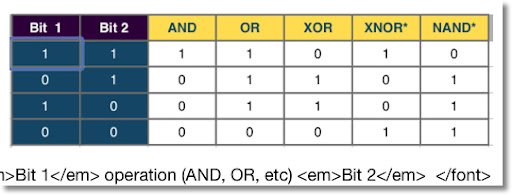How the TI-36X Works With Binary Numbers

All binary numbers work with 10 bit integers. Attempting to work integers greater than 10 bits causes a SYNTAX Error. This error is not mentioned in the TI-36X Manual.

The binary number of a TI-36X looks like this:

##########

where # is a 0 or 1. If you enter less than 10 bits, the calculator "fills" the leftmost bits with 0. Note that I designated the leftmost bit (10th bit) red and bold. This is known as the sign bit. If this bit is 0, then the integer is positive.

(sign) (2^8) (2^7) (2^6) (2^5) (2^4) (2^3) (2^2) (2^1) (2^0)
0 [ 1 = "on", 0 = "off"]

However, if the leftmost bit (10th bit) is 1, then the integer is negative. I am not 100% sure how the rest of the bits are designated.

Note in all examples, I designate binary numbers with the "b", like the calculator does.

10001b = 0000010001b = 17

1111101111b = -17

For the TI-36X Pro, the boundary for binary integers in the interval [-511, 511].
(2^9 - 1 = 511) Remember, the 10th bit is the sign bit on this calculator.

When using logic operators, the TI-36X compares each bit, including the 10th bit.

By the way, binary integers can be stored and recalled in variables.

Examples:

Let x = 0000011101b (5th, 4th, 3rd, and 1st bits are on, x = 29)
and y = 0000010100b (5th and 3rd bits are on, y = 20)

(decimal equivalents in parenthesis)
x and y = 0000010100b (29 and 20 = 20)
x or y = 0000011101b (29 or 20 = 29)
x xor y = 0000001001b (29 xor 20 = 9)
x xnor y = 1111110110b (29 xnor 20 = -10)
x nand y = 1111101011b (29 nand 20 = -21)

So, when I think of logic operations, I think of comparing binary numbers (or binary equivalent of integers) bit by bit, given a designated amount of bits.

Hope this helps,

Eddie.

This blog is property of Edward Shore. © 2012

### Calculus Revisited #11: Integration by Substitution

Welcome to Part 11 of our 21-part Calculus Revisited journey! Today we are continuing with integrals - and starting the first of four blog entries concerning techniques of integration. I will try my best to explain each one but I feel the best way to demonstrate these techniques is by example.

Integration by Substitution

We have the integral ∫ f(x) dx.

Find a function u(x) such that

du = u'(x) dx and

∫ f(u) * du is easier to integrate than ∫ f(x) dx.

Then ∫ f(u) du = F(u) + C

For the definite integral

b
∫ f(x) dx
a

du = u'(x) dx

and the integral is re-written as

u(b)
∫ f(u) du = F(u(b)) - F(u(a))
u(a)

Let's get to the solved problems to demonstrate this technique.

Problems
Indefinite Integrals

1. ∫ √(4 + 5x) dx

Let u(x) = 4 + 5x
Then du = 5 dx

Note that √u = √(4 + 5x)
and dx = du/5

Rewriting the integral:

∫ √(4 + 5x) dx
= ∫ √u * 1/5 du
= 1/5 * ∫ √u du
= 1/5 * (u^(3/2))/(3/2) + C
= 1/5 * 2/3 * u^(3/2) + C
= 2/15 * u^(3/2) + C
When substitution is used, please don't not forget to state the answer in the original variable, in this case, x. u = 4 + 5x
= 2/15 * (4 + 5x)^(3/2) + C

2. ∫ sin x / √(1 + 2 cos x) dx

Let u = 1 + 2 cos x
Then du = - 2 sin x dx

Note - du/2 = sin x dx (which matches the numerator)

Rewriting the integral:
∫ sin x / √(1 + 2 cos x) dx
= ∫ 1/√u * -1/2 du
= -1/2 * ∫ u^(-1/2) du
= -1/2 * u^(1/2) * 2 + C
= -u^(1/2) + C
= -√u + C
Remember... the answer must contain the original variable!
= -√(1 + 2 cos x) + C

The right substitutions make integration much easier. The next two problems illustrate this.

3. ∫ sin^4 x * cos x dx

Let u = sin x
Then du = cos dx (note the cos x in the integral)

∫ sin^4 x * cos x dx
= ∫ u^4 du
= u^5/5 + C
= sin^5 x/5 + C

4. ∫ e^x / (e^(2x) + 1) dx

Let u = e^x
Then du = e^x dx (matches the numerator)

∫ e^x / (e^(2x) + 1) dx
= ∫ du/(u^2 + 1)
= atan u + C
= atan (e^(2x)) + C

Definite Integrals

If you use the substitution technique with indefinite integrals, do NOT forget to account for the limits! For definite integrals, you will recalculate the limits to account for the substitution. The next two problems illustrate this point.

5. Calculate

1
∫ cos (2x) dx
-1

Let u = 2x
Then du = 2 dx

Note du/2 = dx (try to get the term with the dx to match some part of the integral)

Accounting for the limits:
New Upper Limit = 2 * 1 = 2
New Lower Limit = 2 * -1 = -2

Putting it together:

1
∫ cos (2x) dx
-1

2
= ∫ cos u * 1/2 du
-2

= 1/2 * (sin(2) - sin(-2))

Recall sin(-x) = -sin x

= 1/2 * (sin(2) + sin(2)) = sin(2) ≈ 0.90930

Finding the right substitution may be tricky at times. Algebraic manipulation may be necessary. Here is a more difficult problem.

6. Calculate

9
∫ √t / (√t - 1) dt
4

Let u = √t - 1
Then du = 1/(2√t) dt

To help match the integral, (2√t) du = dt

Note that 2√t = 2(u + 1) = 2u + 2 (Look at the substitution and solve for √t).

So (2u + 2) du = dt

We can't forget about the limits, since we are working with a definite integral.
u = √t - 1

New Upper Limit: √9 - 1 = 4 - 1 = 3
New Lower Limit: √4 - 1 = 2 - 1 = 2

Putting this together:

9
∫ √t / (√t - 1) dt
4

2
= ∫ (u + 1)/u * (2u + 2) du
1

2
= ∫ (1 + 1/u) * (2u + 2) du
1

2
= ∫ 2u + 2 + 2 + 2/u du
1

2
= ∫ 2u + 4 + 2/u du
1

The antiderivative is u^2 + 4u + 2 ln u

= ( (2)^2 + 4(2) + 2 ln (2) ) - ( (1)^2 + 4(1) + 2 ln (1) )

= ( 4 + 8 + 2 ln 2 ) - ( 1 + 4 + 2 * 0 )

= 7 + 2 ln 2 ≈ 8.38629

Best thing to do if you are in a calculus course is to practice, practice, practice!

I hope these six example problems give you an understanding of the substitution technique. Mastery of this technique will come with time and practice - and will speed up integral evaluation.

Next time, we look at the famous (or maybe infamous) Integration by Parts technique.

See you soon,

Eddie

This blog is property of Edward Shore. © 2012

## Wednesday, May 2, 2012

### Link to Part 10: Calculus Revisited

Here is the link to Part 10 of our Calculus Revisited Series: Integration Basics

http://edspi31415.blogspot.com/2012/04/calculus-revisited-10-integration.html

(The original post is buried in April 2012)

## Tuesday, May 1, 2012

### First Look: Casio FC-200V Financial Calculator (Updated 4/6/2014)

Hi everyone! In tonight's blog entry we will look at the Casio FC-200V solar financial calculator. I purchased this calculator from Amazon on Super Saver shipper and it came one day earlier than scheduled. It is a rare calculator in terms that I believe it is the only financial calculator besides the Texas Instruments BA-35 Solar that is to be solar-powered. (I could be wrong and if I am feel free to let me know.)

Below is the picture of the calculator in it's packaging: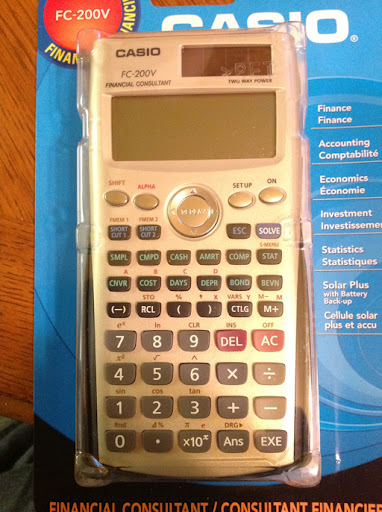Packaging
The packaging came with the calculator, a hard case with a cheat sheet, and three instruction manuals: one in Spanish, one in French, and one in English.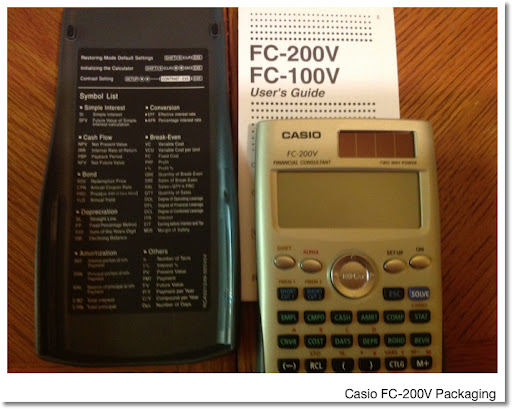Features

From the packaging, the FC-200V is said to have the following:

* SMPL (Simple Interest)
* CMPD (Compound Interest)
* CASH (Cash Flows: NPV, NFV, PBP, and IRR)**
* AMRT (Amortization)
* DPRC (Depreciation: SL, Fixed %, SYD, DB)
* Bonds
* BEVN (Break Even)
* DAYS (Days & Days Between Dates)
* COST (Cost Selling Margin)

Comparing the FC-200V against other financial calculators (I'll use the Hewlett Packard HP 10bII+ for comparison tonight - picture shown below), it seems that the FC-200V breaks away from the traditional financial calculator set up.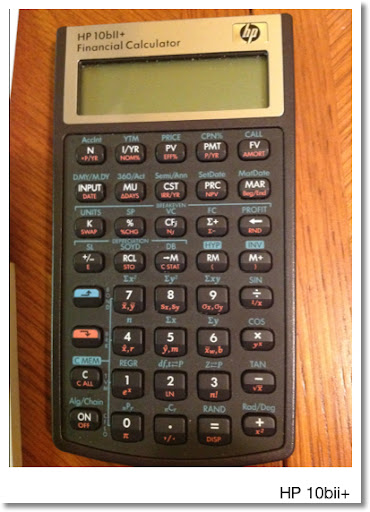Time Value of Money
Most financial calculators sport five keys for using TVM (Time Value of Money) calculations:

N = number of periods
I/YR = yearly interest rate (some have I for periodic interest rate instead)
PV = present value
PMT = periodic payment
FV = future value

The FC-200V breaks away from this model and instead presents TVM calculations in two modules:
1. SMPL for Simple Interest
2. CMPD for Compound Interest

SMPL

Pressing SMPL gives the following options:
Set: Toggle between 365 or 360 days a year
Days: Number of Days
I%: Annual Interest Rate
PV: Present Value

This mode solves for SI (Simple Interest) and SFV (Simple Future Value).

Note: All my examples, unless stated otherwise, use 365 days per year.

Example: If you deposit \$900.00 in the bank today in an aggressive 90-day term CD that pays 5% a year, how much will you have in 90 days?

SMPL Mode:
Set: 365
Days = 90
I% = 5
PV = -900

Results:
SI = 11.10
SFV = 911.10

You could use the TVM keys, however, you answers match better if you use the formula:

SFV = -(PV + Days/365 * I%/100 * PV) (denominator can be 360)

CMPD Mode
This is the traditional TVM set up:
Set*: End or Beginning
n = Number of compounding periods
I% = Annual Interest Rate
PV = Present Value
PMT = Periodic Payment
FV = Future Value
P/Y = Number of payments per year (affects n)
C/Y = Number of compounding periods per year (affects interest) - set separately from P/Y

Use the SOLVE key to solve for the desired variable. Use the cash flow: inflows (receipts) are positive, outflows (disbursements) are negative.

FC-200V vs. HP 10bII+

Example 1: Solving for Payment - END Mode
N = 48, I%YR = 9%, PV = 60,000, FV = 0, P/Y = C/Y = 12

PMT = -1,493.10 (HP 10bII+ is instant, beating out FC-200V by a microsecond)

Example 2: Solving for Interest - END Mode
N = 60, PV = -10,000, PMT = -250, FV = 30,000, P/Y = C/Y = 12

I%YR = 5.15% (The HP 10bii+ being operated by battery only, returns this result instantly. However, the FC-200V returns the result within 3 seconds, so it is not a slow poke - not bad for a solar calculator)
Cash Flow**

The FC-200V stores up to 80 single cash flows, or 40 when frequency is involved. By contrast, the HP 10bII+ can store up to 45 cash flows.

Like the HP10bII+, the FC-200V can solve for NPV (Net Present Value), NFV (Net Future Value), and IRR (Internal Rate of Return). The FC-200V also solves for PBP (Payback Period).

Example:
Cash Flows for a 5 year project:
Initial Investment (x1): -\$35,000
Year 1 (x2): \$10,000
Year 2 (x3): \$12,000
Year 3 (x4): \$16,600
Year 4 (x5): \$17,500
Year 5 (x6): \$24,100

Use a discount rate of 10%

To enter cash flows, hit the [CASH] key and select Csh row. It will take you to the stats screen. Yes, the FC-200V shares the statistic list with the cash flow list.

NPV = \$23,397.03
NFV = \$37,681.15
IRR (takes a few seconds on the FC-200V) = 29.96%

** On the HP 10bii+: don't forget rate goes I/YR and P/YR = 1

<hr>UPDATE:  4/6/2014

It doesn't look like the FC-200V can handle certain types of cash flow problems correctly.  We run into trouble when at least one flow CFn has the same sign as the initial flow (designated as CF1 on the FC-200V).  Please see the comments below for examples.

Hence, if you are looking for a reliable cash flow function, pass on the FC-200V.

I apologize for any inconvenience. - Eddie

<hr>

Other Features
CNVR: Convert between EFF (effective) and APR (nominal percentages) with compounding

n: Payments per year
I%: Interest to be converted, select to EFF or to APR

The [COMP] key returns you to the home screen.

COST: Cost-Sell-Margin

DAYS: Days between dates using 360 day years or 365 day years. Use the format for dates: mmddyyyy (USA) or ddmmyyyy (International) (no decimal point)

Bonds and Depreciation

An Expansive Breakeven Module, including Degree of Operating Leverage, Degree of Financial Leverage, Degree of Combined Leverage, and Margin of Safety

Statistics

The FC-200V has the same statistical regressions of its scientific sisters fx-115ES and fx-300ES: linear, logarithmic, two types of exponential, power, inverse, quadratic.

Scientific Functions

On recent financial calculators, there has been a growing number of scientific functions. The FC-200V is no exception. Trigonometry (inverse functions have to be accessed by the catalog), polar and rectangular conversions (via the catalog), powers, roots (real numbers only), logarithms, exponentials, hyperbolic functions (via the catalog), and random numbers. No normal distribution functions though. However, room for two shortcuts and formula memories.

I will looking through the manual over the next week. This is just a quick overview of the FC-200V.

This blog is property of Edward Shore. © 2012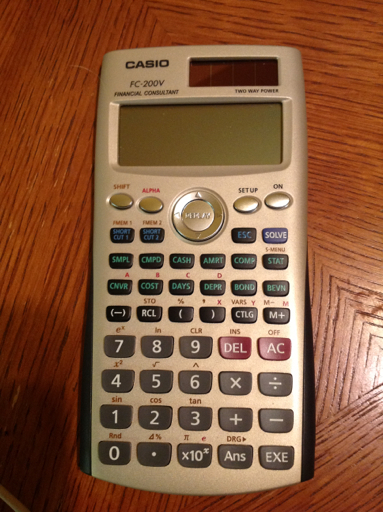### Links to the First Nine Parts of Calculus Revisited

#1: Functions
http://edspi31415.blogspot.com/2012/04/calculus-revisited-1-functions.html

#2: Trig Functions
http://edspi31415.blogspot.com/2012/04/calculus-revisited-2-working-with.html

#3: Logarithm and Exponential Functions
http://edspi31415.blogspot.com/2012/04/calculus-revisited-3-logarithmic-and.html

#4: Limits
http://edspi31415.blogspot.com/2012/04/calculus-revisited-4-limits.html

#5: Derivatives
http://edspi31415.blogspot.com/2012/04/calculus-revisited-5-derivatives.html

#6: Chain Rule
http://edspi31415.blogspot.com/2012/04/calculus-revisited-6-chain-rule.html

#7: Finding Extrema
http://edspi31415.blogspot.com/2012/04/calculus-revisited-7-finding-extrema.html

#8: Related Rates
http://edspi31415.blogspot.com/2012/04/calculus-revisited-8-related-rates.html

#9: Newton's Method
http://edspi31415.blogspot.com/2012/05/calculus-revisited-9-newton-method.html

### Calculus Revisited #9: Newton's Method

Welcome to blog entry #9 of 21 of our Calculus Revisited. Today we will cover Newton's Method: an effective and powerful way of finding roots of functions.

Process: Newton's Method

Goal: Find roots of the function f(x). That is, solve for x: f(x) = 0

2. Calculate x_(n+1) = x_n - f(x_n)/f'(x_n) (function over its derivative)

3. If x_n+1 matches x_n, you are done, with the root being x_n+1. You determine how accurate the answer is by selecting how many decimal points to match. Calculator accuracy (what you see on the screen) varies from 10 to 12 decimal points.

Caution: The choice of initial value is important. Usually, selecting initial guesses close to the root works the best, but this does not always work.

Also, this method is not 100% in finding roots. If you get x_n and x_n+1 flopping between two distinct values, you are caught in a loop - a root will not be found.

I recommend that you use a calculator when working with Newton's Method. On scientific calculators, you may be able to take advantage of the last answer feature, setting x_n = ans.

Example: (TI -36X Pro, TI-30 Multiview, Casio fx-300, Casio fx-115ES, most graphing calculators)
2 ENTER (2 is stored in ans)
ans - f(ans)/f'(ans) (calculates x_1 with x_n = 2)
Keep pressing ENTER

Problems
Newton's Method will often be used to estimate nth roots (square root, cube root, etc). The first problem will be an example of such.

1. Estimate √6 to 5 decimal places.

Observe that √4 = 2 and √9 = 3, which means √6 lies in between 2 and 3. Let's make an initial guess 2.5.

Use the function f(x) = x^2 - 6. Why?

x^2 = 6
x^2 - 6 = 0

Finding the root to the above equation leads to √6.

Then f'(x) = 2x and

x_n+1 = x_n - (x^2 - 6)/(2x)

Having x_0 as the initial guess,
x_0 = 2.50000
x_1 = 2.45000
x_2 = 2.44949
x_3 = 2.44949

(x_1 = 2.5 - (2.5^2 - 6)/(2*2.5) = 2.45,
x_2 = 2.45 - (2.45^2 - 6)/(2*2.45) = 2.44949, and so on)

So to five decimal places, √6 ≈ 2.44949

2. Find a root of the equation e^x - x = 5 for any x > 0. Let x_0 = 2. (the initial guess)

Get the equation in form of f(x) = 0:
e^x - x = 5
e^x - x - 5 = 0

Then f(x) = e^x - x - 5, f'(x) = e^x - 1, and

x_n+1 = x_n - (e^x_n - x_n - 5)/(e^x_n - 1)

By calculation, we get:

x_0 = 2.00000
x_1 = 1.93911
x_2 = 1.93685
x_3 = 1.93685

Then a root of f(x) is x≈1.93685.

3. Solve x^4 - 4x - 4 = 0 with the condition -1 < x < 1. Find the root to five decimal places.

Hint: You may want to graph f(x) to estimate an appropriate initial guess.

Let x0 = -.5

Then f(x) = x^4 - 4x - 4, f'(x) = 4x^3 - 4, and

x_n+1 = x_n - (x_n^4 - 4x_n - 4)/(4x_n^3 - 4)

By calculation, we get:
x_0 = -0.50000
x_1 = -0.93056
x_2 = -0.86520
x_3 = -0.86198
x_4 = -0.86198

A root of f(x) where -1
Here is a situation where Newton's Method does not work for three picks. Not all f(x) will be successful.
4. Find a root of f(x) = √(x^2 - 4) Fortunately, we can find them using algebraic methods (x = ± 2).

f(x) = √(x^2 - 4)
f'(x) = 1/(2 * √(x^2 - 4) * 2x = x/√(x^2 - 4)
x_n+1 = x_n - (x^2 - 4)/x

If x_0 = 1, then subsequent calculations result in a loop between 1 and 4.
If x_0 = 1.5, then subsequent calculations result in a loop between 1.5 and 2.66667.
If x_0 = 1.75, then subsequent calculations result in a loop between 1.75 and 2.828571.

You may try this with other x_0 and run into similar trouble.

Just be aware.

That wraps it up on Newton's Method. Next we head into integration! - Eddie

This blog is property of Edward Shore. © 2012

### HP 15C: Error Function and Lower Tail Normal Cumulative Function

HP 15C:   Error Function and Lower Tail Normal Cumulative Function Formulas Used  Error Function  erf(x) = 2 ÷ √π * ∫( e^(-t^2) dt, t = 0 t...